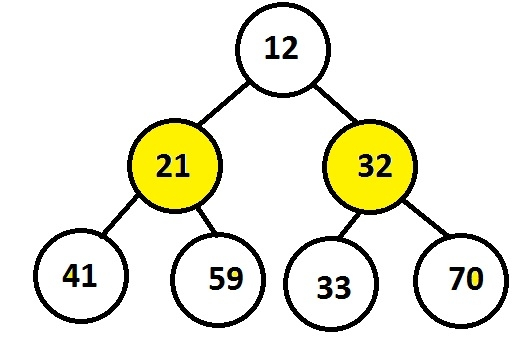# Print middle level of perfect binary tree without finding height in C language

The program should print the middle level of a binary tree e.g. if there are 4 levels in a binary tree than the program must print the level 2 nodes but the demand here is to calculate the level without finding the height.

Perfect binary tree is a tree in which interior nodes must have two children and all leave nodes should be at the same level or depth.Here,

• Interior nodes 21 and 32 both are having children

• Leaf nodes are 41, 59, 33 and 70 all lies at the same level.

Since it is satisfying both the properties it’s a perfect binary tree.

## Example

Input : 12 21 32 41 59 33 70
Output : 21 32

The approach used here is just like finding middle elements of a linked list by checking the left and right pointer of a node i.e. whether NULL or NOT NULL by making a recursive call to a function.

The below code shows the c implementation of the algorithm given.

## Algorithm

START
Step 1 -> create node variable of type structure
Declare int key
Declare pointer of type node using *left, *right
Step 2 -> create function for inserting node with parameter as value
Declare temp variable of node using malloc
Set temp->data = value
Set temp->left = temp->right = NULL
return temp
step 3 - > Declare Function void middle(struct Node* a, struct Node* b)
IF a = NULL||b = NULL
Return
IF ((b->left == NULL) && (b->right == NULL))
Print a->key
Return
End
Call middle(a->left, b->left->left)
Call middle(a->right, b->left->left)
Step 4 -> Declare Function void mid_level(struct Node* node)
Call middle(node, node)
Step 5 -> In main()
Call New passing value user want to insert as struct Node* n1 = New(13);
Call mid_level(n1)
STOP

## Example

#include <stdio.h>
#include<stdlib.h>
struct Node {
int key;
struct Node* left, *right;
};
struct Node* New(int value) {
struct Node* temp = (struct Node*)malloc(sizeof(struct Node));
temp->key = value;
temp->left = temp->right = NULL;
return (temp);
}
void middle(struct Node* a, struct Node* b) {
if (a == NULL || b == NULL)
return;
if ((b->left == NULL) && (b->right == NULL)) {
printf("%d ",a->key);
return;
}
middle(a->left, b->left->left);
middle(a->right, b->left->left);
}
void mid_level(struct Node* node) {
middle(node, node);
}
int main() {
printf("middle level nodes are : ");
struct Node* n1 = New(13);
struct Node* n2 = New(21);
struct Node* n3 = New(44);
struct Node* n4 = New(98);
struct Node* n5 = New(57);
struct Node* n6 = New(61);
struct Node* n7 = New(70);
n2->left = n4;
n2->right = n5;
n3->left = n6;
n3->right = n7;
n1->left = n2;
n1->right = n3;
mid_level(n1);
}

## Output

If we run above program then it will generate following output.

middle level nodes are : 21 44

Updated on: 22-Aug-2019

118 Views